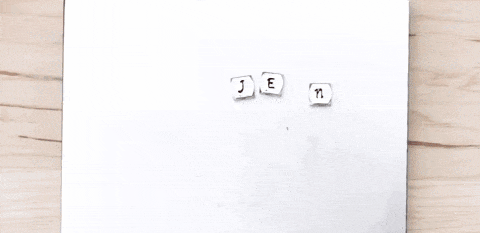IntroductionIntroductionINTRO
We can have all sorts of wacky beads or charms, but no matter what, their holes have to line up in order to add and remove them!Our decimal points are like the bead holes. We might have decimals of different lengths, but as long as we make sure to line up our decimal points, we’ll be able to add and subtract them up with no problem!
Try dragging the bottom decimal to line up the decimal points!Check out our
Calculator
or explore our
Lesson
and
Practice

CALCULATOR

KEY STEPS

## How to Add and Subtract Decimals

### Step 1. Line up the decimal points.

We have to line up the decimal points just like we have to line up the holes in beads to make a bracelet.

### Step 2. Add trailing zeros.

This step is optional, but we can add 's to the end of our shorter decimal to make adding and subtracting decimals easier.

The decimal point in the final answer should line up with the other decimal points.
LESSON

Adding and subtracting decimals is just like adding and subtracting whole numbers but with an additional twist: we have to make sure we line up our decimal points!
Try dragging the bottom decimal to line up the decimal points!Once we do that, we can add trailing zeros to the ends of the decimals to line everything up without changing any values.

### Trailing Zeros 🚶🏿🚶🏼🚶🏾

Trailing zeros are polite and never cut in the middle of a decimal. They always go at the end.
So we can do this:
But we CANNOT do this:
⛔️

PRACTICE

## Practice: Adding and Subtracting Decimals

Question 1 of 10:

### Step 1. Line up the decimal points.

We have to line up the decimal points just like we have to line up the holes in beads to make a bracelet.CONCLUSION
Incredible job, look at you go! Thanks for checking out this lesson ☺️🙏. Where to next?Leave Feedback